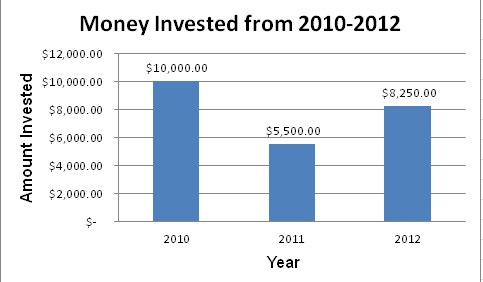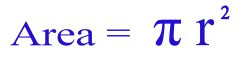# Complex Images

## Charts or Graphs

Usually a graph on a webpage is an image file placed using html code. As such, an appropriate Alt tag should be included. However, on its own, an alt tag doesn’t do a graph justice.

The following bar graph with the alt tag alt=”graph of money invested since 2010” does not convey all the information represented by the graph.In order to serve a wider audience and improve accessibility of a graph it is wise to include a text summary (perhaps describing trends) and a properly coded table (see Table section) of the data placed near the graph.

The improved graph has a title, axis labels, data labels, alt text, a summary, and table of data.Summary:

The bar graph shows that every year between and including 2010 and 2012, the money that has been invested has decreased 50%.

Data Table

Amount Year
\$10,000 2010
\$8500 2011
\$5000 2012

## Infographics

These images can hold massive quantities of information in a visual way. For a person who is blind or with low vision these images are troublesome. The information contained within an infographic is too large for an Alt tag. Therefore, a text equivalent is required either below the image or on a separate web page.

## Flowcharts

Another type of graphic is a flowchart or concept map that shows relationships between ideas or concepts. Providing a text based outline of the diagram assists with accessibility.

## Math Equations

Equations and scientific notations can be handled by using images with accompanied Alt tags for simple less complex equations to using MathML for the complex. MathML is an XML markup language designed to display complex mathematical expressions (e.g. fractions, square roots, matrices, bounded integrals). The theoretical advantage of MathML is that equations are represented as text instead of images.

### Using Images and Alt tags

If your website used an image for the formula to calculate the area of a circle it might look like the following:For someone using a screen reader they have no idea whether the image contains a picture or a formula. Since the formula is simple in nature an Alt tag would probably suffice. The alternative text for a formula can be written in Nemeth MathSpeak for anyone who has learned that system.

Alt=”formula for area of a circle. Area equals pi dot radius sup 2”

### MathML

To create MathML code it is best to use to use an equation editor such as:

• MathType
• MathEQ Expression Editor
• MathMagic Personal Edition
• Scientific NoteBook Scientific
• Fire Math

One of the more common is MathType which can integrate with MS Office. Please see “External Resources” for more information and step-by-step instructions on using MathType.

## Queen's Web Standards and Accessibility Development Guide (WSADG)

For your reference, sections of the WSADG used for this part of the tutorial are: